Courses

# Value Based Questions- Triangles Class 9 Notes | EduRev

## Class 9 Mathematics by Full Circle

Created by: Full Circle

## Class 9 : Value Based Questions- Triangles Class 9 Notes | EduRev

The document Value Based Questions- Triangles Class 9 Notes | EduRev is a part of the Class 9 Course Class 9 Mathematics by Full Circle.
All you need of Class 9 at this link: Class 9

Question 1. In a group-discussion on “Save Water” five students Shagun, Preeti, Deepak, Nitin and Piyush are possitioned at A, B, C, X and Y respectively as shown in the following figure such that AB = AX = BX and AC = AY and CY. Also, ΔABC is an equilateral triangle.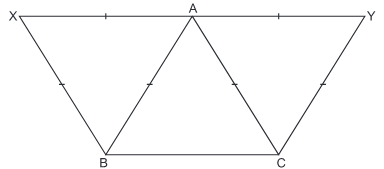(i) Show that CX = BY.

(ii) Which mathematical concept is used in the above problem?

(iii) By arranging a group discussion on ‘save-water’, which values are depicted by the school?
Solution.
(i) Join BY and CX.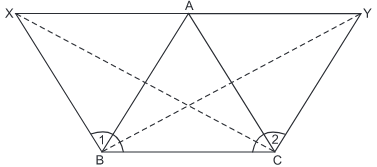∵ ΔABC is an equilateral Δ
∴ AB = BC = AC                     ..... (1)
But AB = AX = BX                     ..... (2)
Also AC = AY = CY                     ..... (3)

∴ From (1), (2) and (3), we have:
BX = CY ..... (4)
Since, AB = AX = BX                    [Given]
∴ ΔABX is an equilateral Δ
⇒ ∠ABX = 60° Similarly ∠ACY = 60°
Also ∠ABC = ∠ACB = 60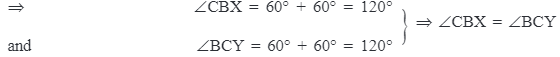Now, In ΔCBX and ΔBCY, we have BX = CY                    [from 4]
∠CBX = ∠BCY                     [Proved above]
BC = BC                    [Common]
∴ ΔCBX ≌ΔBCY
⇒ CX = BY
(ii) Triangles                    [Geometry].
(iii) Inculcating discussion ability and developing an attitude of saving natural resources.

Question 2. Rajat and Shankar live together at B. Their work places are at A and C respectively, such that A, B and C form a triangle. BD, the bisector of ∠ABC, meets AC at right angle. Various distances are expressed in terms of x and y as shown in the following figure.
They use bicycles instead of cars to go to their work places:
(i) Find the distance between ‘A’ and ‘C’.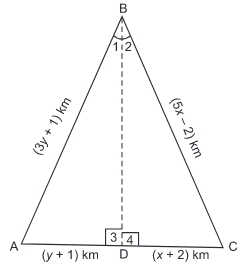(ii) Which mathematical concept is used in the above problem?
(iii) By using bicycles instead of cars, which values are depicted by Rajat and Shankar?
Solution.
(i) Since, BD is perpendicular to AC
∴ ∠3 = ∠4 = each 90°                     ..... (1)

Also, BD is bisector of ∠ABC,
∴ ∠1 = ∠2
Now, in ΔsABD and CBD,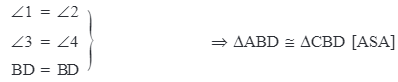∴ AB = CB and AD = CD
or 3y + 1 = 5x - 2
⇒ 3y - 5x = ∠3 and y + 1 = x + 2
⇒ y - x = 1

Solving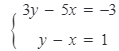we get x = 3, y = 4
Now distance between A and C  = AD + DC
=[y + 1] km + [x + 2] km
= [4 + 1] km + [3 + 2] km
= 5 km + 5 km = 10 km

(ii) Triangles                    [Geometry].

(iii) Following value factors are depicted:
(a) Environment protection as bicycles are pollution-free.
(b) Physical exercise for good health.
(c) Saving energy as bicycles do not consume petrol.

132 docs

,

,

,

,

,

,

,

,

,

,

,

,

,

,

,

,

,

,

,

,

,

;